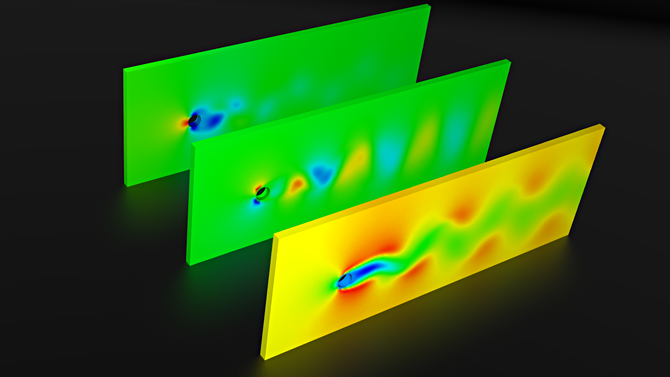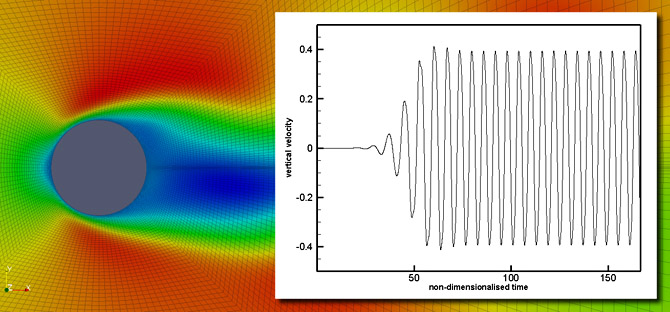E-Mail: info@zetacomp.com

# Benchmark Data (3d)

Classical benchmarks, as outlined in the 7th Edition of The Finite Element Method For Fluid Dynamics, are provided for both verification of the numerical methods and also as tutorial examples. The accompanying programs can be found in the Software section of the website. Both the source code and some pre-compiled executables are available for the 2d versions. The full CBSFlow program must be purchased in order to acquire the source code.

### 3D Lid-driven Cavity

This is a 3d variant of the classic benchmark for incompressible Navier-Stokes solvers. The domain is a unit square box in the XZ plane, with a Y-length of 0.1. The domain comprises 3 no-slip walls (code 500), and the top "wall" moving with a unit horizontal velocity,no vertical component (code 503). The additional faces (Y=0 and Y=0.1) are denoted as symmetric (code 505). The lid is non-leaky (at the top corner nodes, u=0,v=0,w=0 is prescribed). To determine the relative pressure field, a single prescibed value may be needed (semi-implicit only). In which case, P=0 is applied at node 1. This is a forced convection problem.

The Input zip file contains only the files necessary to run the specified program, the All zip file also contains the program output. Results are given for a Reynolds number of 100.

CBSFlow (2D/3D). The non-uniform mesh: 8000 linear tetrahedral elements, 2205 nodes.

CBSFlow (2D/3D). The non-uniform mesh: 1600 linear hexahedral elements, 2205 nodes.

### 2D/3D flow around a cylinder

This problem is a popular test case for validating the transient part of numerical schemes. A typical geometry and associated boundary conditions can be seen below. The computational domain is defined based upon the cylinder diameter. The Strouhal number for this problem is approximately 0.167. However, this value is impacted by the extent of the computational domain (Behr, Hastreiter, Mittal and Tezduyar Comput Methods Appl Mech Eng 1995 123:309-316).

The 3d mesh provided contains 69948 linear tetrahedral elements. This problem requires a long temporal domain, to establish regular vortex shedding. A non-dimensional real time of between 150-200 is typical, with the real time stepsize itself less than 0.16666. Due to the 15D exterior boundary offset of the 2d mesh, a Strouhal number of 0.166 was obtained. The figure below shows the qualitative values of velocity (x and z components) and pressure, with U foremost and P rearmost.The 2d mesh is a non-uniform structured mesh. Extra refinement is required both around the cylinder and in the wake, in order to accurately capture vortex shedding, drag and lift. The vertical velocity at (11.0,0.0) is presented for a time domain which extended to a non-dimensional time of 166. The real time step was 0.0208333. Some selected results can be downloaded using the above links.